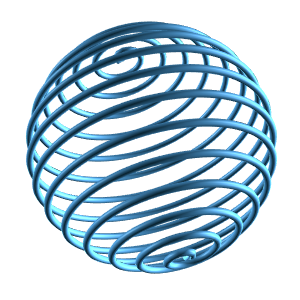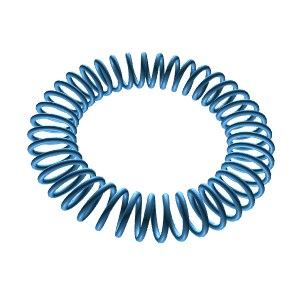### Free Ginckers

#### Tube: Cone

Template: Graphics/3d-tube-shape

Discription: This template creates a 3D cone tube that extrudes along a 3D curve based on a set of parametric equations x(t), y(t), and z(t).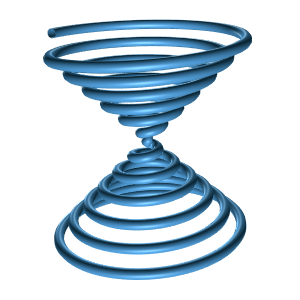#### Tube: Figure Eight Knot

Template: Graphics/3d-tube-shape

Discription: This template creates a 3D figure eight knot tube that extrudes along a 3D curve based on a set of parametric equations x(t), y(t), and z(t).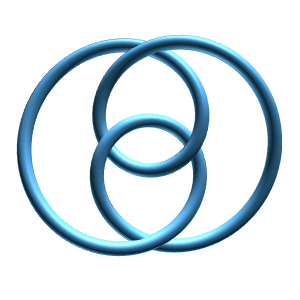#### Tube: Figure Eight knot 2

Template: Graphics/3d-tube-shape

Discription: This template creates another figure eight knot tube that extrudes along a 3D curve based on a set of parametric equations x(t), y(t), and z(t).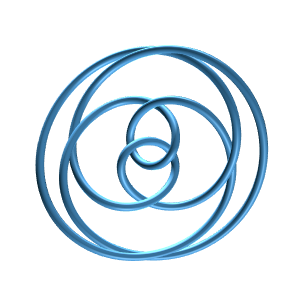#### Tube: Figure Eight knot 3

Template: Graphics/3d-tube-shape

Discription: This template creates yet another figure eight knot tube that extrudes along a 3D curve based on a set of parametric equations x(t), y(t), and z(t).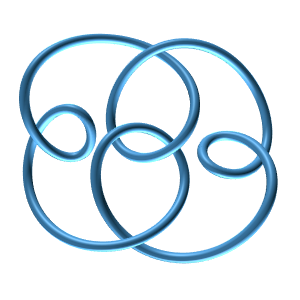#### Tube: Heart

Template: Graphics/3d-tube-shape

Discription: This template creates a heart tube that extrudes along a 3D curve based on a set of parametric equations x(t), y(t), and z(t).#### Tube: Helix

Template: Graphics/3d-tube-shape

Discription: This template creates a helix tube that extrudes along a 3D curve based on a set of parametric equations x(t), y(t), and z(t).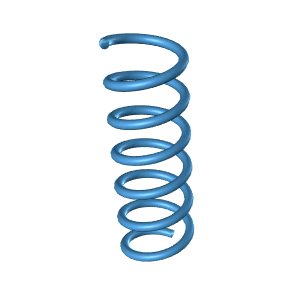#### Tube: Polynomial Knot (3,1)

Template: Graphics/3d-tube-shape

Discription: This template creates a polynomial (3,1) knot tube that extrudes along a 3D curve based on a set of parametric equations x(t), y(t), and z(t).#### Tube: Polynomial Knot (4,1)

Template: Graphics/3d-tube-shape

Discription: This template creates a polynomial (4,1) knot tube that extrudes along a 3D curve based on a set of parametric equations x(t), y(t), and z(t).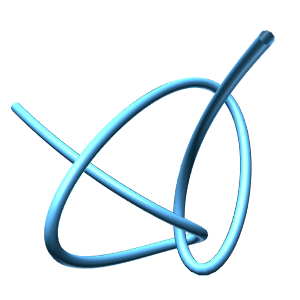Template: Graphics/3d-tube-shape

Discription: This template creates a slinky tube that extrudes along a 3D curve based on a set of parametric equations x(t), y(t), and z(t).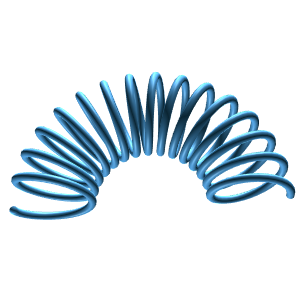#### Tube: Sphere

Template: Graphics/3d-tube-shape

Discription: This template creates a sphere tube that extrudes along a 3D curve based on a set of parametric equations x(t), y(t), and z(t).